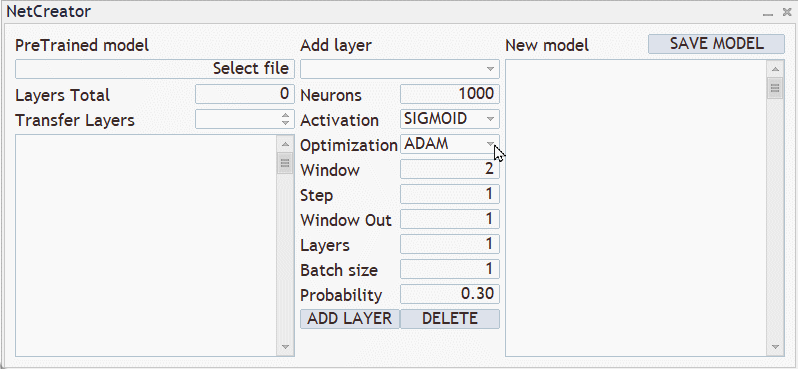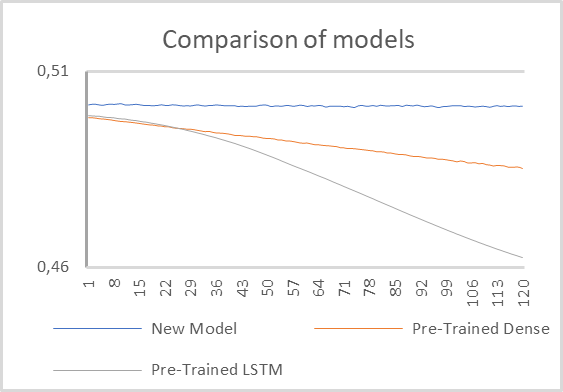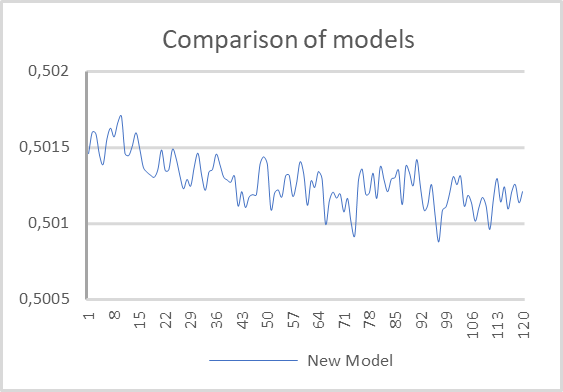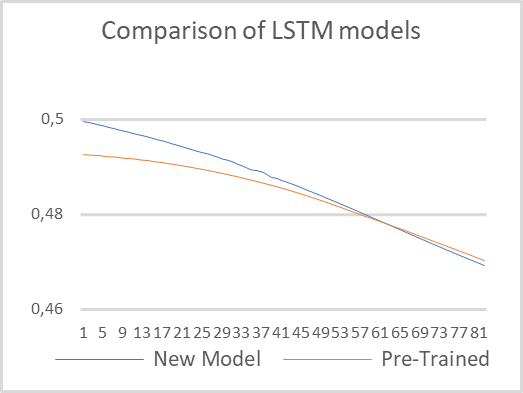# 神经网络变得轻松（第二十五部分）：实践迁移学习

• 概述
• 1. 一般测试的准备问题
• 2. 为测试创建智能系统
• 3. 为测试创建模型
• 4. 测试结果
• 结束语
• 参考文献列表
• 本文中用到的程序

### 2. 为测试创建智能系统

• NeuroNet.mqh — 我们创建神经网络的函数库
• SymbolInfo.mqh — 访问交易品种数据的标准库
• Oscilators.mqh — 协同振荡器操作的标准库

`//+------------------------------------------------------------------+  //| Includes                                                         |  //+------------------------------------------------------------------+  #include "....NeuroNet_DNGNeuroNet.mqh"  #include <TradeSymbolInfo.mqh>  #include <IndicatorsOscilators.mqh>  //---  enum ENUM_SIGNAL    {     Sell = -1,     Undefine = 0,     Buy = 1    };  `

`//+------------------------------------------------------------------+  //|   input parameters                                               |  //+------------------------------------------------------------------+  input int                  StudyPeriod =  2;            //Study period, years  input string               FileName = "EURUSD_i_PERIOD_H1_test_rnn";  ENUM_TIMEFRAMES            TimeFrame   =  PERIOD_CURRENT;  //---  input group                "---- RSI ----"  input int                  RSIPeriod   =  14;            //Period  input ENUM_APPLIED_PRICE   RSIPrice    =  PRICE_CLOSE;   //Applied price  //---  input group                "---- CCI ----"  input int                  CCIPeriod   =  14;            //Period  input ENUM_APPLIED_PRICE   CCIPrice    =  PRICE_TYPICAL; //Applied price  //---  input group                "---- ATR ----"  input int                  ATRPeriod   =  14;            //Period  //---  input group                "---- MACD ----"  input int                  FastPeriod  =  12;            //Fast  input int                  SlowPeriod  =  26;            //Slow  input int                  SignalPeriod =  9;            //Signal  input ENUM_APPLIED_PRICE   MACDPrice   =  PRICE_CLOSE;   //Applied price    `

`CSymbolInfo          Symb;  CNet                 Net;  CBufferFloat        *TempData;  CiRSI                RSI;  CiCCI                CCI;  CiATR                ATR;  CiMACD               MACD;  CBufferFloat         Fractals;    `

`uint                 HistoryBars =  40;            //Depth of history  MqlRates             Rates[];  float                dError;  float                dUndefine;  float                dForecast;  float                dPrevSignal;  datetime             dtStudied;  bool                 bEventStudy;    `

`//+------------------------------------------------------------------+  //| Expert initialization function                                   |  //+------------------------------------------------------------------+  int OnInit()    {  //---     ResetLastError();     if(!Net.Load(FileName + ".nnw", dError, dUndefine, dForecast, dtStudied, false))       {        printf("%s - %d -> Error of read %s prev Net %d", __FUNCTION__, __LINE__, FileName + ".nnw", GetLastError());        return INIT_PARAMETERS_INCORRECT;       }    `

`   if(!Net.GetLayerOutput(0, TempData))        return INIT_FAILED;     HistoryBars = TempData.Total() / 12;     Net.getResults(TempData);     if(TempData.Total() != 3)        return INIT_PARAMETERS_INCORRECT;    `

`   if(!Symb.Name(_Symbol))        return INIT_FAILED;     Symb.Refresh();       if(!RSI.Create(Symb.Name(), TimeFrame, RSIPeriod, RSIPrice))        return INIT_FAILED;       if(!CCI.Create(Symb.Name(), TimeFrame, CCIPeriod, CCIPrice))        return INIT_FAILED;       if(!ATR.Create(Symb.Name(), TimeFrame, ATRPeriod))        return INIT_FAILED;       if(!MACD.Create(Symb.Name(), TimeFrame, FastPeriod, SlowPeriod, SignalPeriod, MACDPrice))        return INIT_FAILED;    `

`   bEventStudy = EventChartCustom(ChartID(), 1, (long)MathMax(0, MathMin(iTime(Symb.Name(), PERIOD_CURRENT,                                    (int)(100 * Net.recentAverageSmoothingFactor * (dForecast >= 70 ? 1 : 10))), dtStudied)),                                      0, "Init");  //---     return(INIT_SUCCEEDED);    }    `

`//+------------------------------------------------------------------+  //| Expert deinitialization function                                 |  //+------------------------------------------------------------------+  void OnDeinit(const int reason)    {  //---     if(CheckPointer(TempData) != POINTER_INVALID)        delete TempData;    }    `

`//+------------------------------------------------------------------+  //| ChartEvent function                                              |  //+------------------------------------------------------------------+  void OnChartEvent(const int id,                    const long &lparam,                    const double &dparam,                    const string &sparam)    {  //---     if(id == 1001)        Train(lparam);    }    `

`void Train(datetime StartTrainBar = 0)    {     int count = 0;  //---     MqlDateTime start_time;     TimeCurrent(start_time);     start_time.year -= StudyPeriod;     if(start_time.year <= 0)        start_time.year = 1900;     datetime st_time = StructToTime(start_time);     dtStudied = MathMax(StartTrainBar, st_time);     ulong last_tick = 0;    `

`   double prev_er = DBL_MAX;     datetime bar_time = 0;     bool stop = IsStopped();    `

`   int bars = CopyRates(Symb.Name(), TimeFrame, st_time, TimeCurrent(), Rates);     if(!RSI.BufferResize(bars) || !CCI.BufferResize(bars) || !ATR.BufferResize(bars) || !MACD.BufferResize(bars))       {        ExpertRemove();        return;       }     if(!ArraySetAsSeries(Rates, true))       {        ExpertRemove();        return;       }     RSI.Refresh(OBJ_ALL_PERIODS);     CCI.Refresh(OBJ_ALL_PERIODS);     ATR.Refresh(OBJ_ALL_PERIODS);     MACD.Refresh(OBJ_ALL_PERIODS);    `

• prev_er — 前一个世代的模型误差
• stop — 生成由用户终止程序的事件
`   MqlDateTime sTime;     int total = (int)(bars - MathMax(HistoryBars, 0) - 300);     do       {        prev_er = dError;        stop = IsStopped();    `

`      for(int it = total; it > 1 && !stop; t--)          {           TempData.Clear();           int i = it + 299;           int r = i + (int)HistoryBars;           if(r > bars)              continue;    `

`         for(int b = 0; b < (int)HistoryBars; b++)             {              int bar_t = r - b;              float open = (float)Rates[bar_t].open;              TimeToStruct(Rates[bar_t].time, sTime);              float rsi = (float)RSI.Main(bar_t);              float cci = (float)CCI.Main(bar_t);              float atr = (float)ATR.Main(bar_t);              float macd = (float)MACD.Main(bar_t);              float sign = (float)MACD.Signal(bar_t);              if(rsi == EMPTY_VALUE || cci == EMPTY_VALUE || atr == EMPTY_VALUE || macd == EMPTY_VALUE || sign == EMPTY_VALUE)                 continue;              //---              if(!TempData.Add((float)Rates[bar_t].close - open) || !TempData.Add((float)Rates[bar_t].high - open) ||                 !TempData.Add((float)Rates[bar_t].low - open) || !TempData.Add((float)Rates[bar_t].tick_volume / 1000.0f) ||                 !TempData.Add(sTime.hour) || !TempData.Add(sTime.day_of_week) || !TempData.Add(sTime.mon) ||                 !TempData.Add(rsi) || !TempData.Add(cci) || !TempData.Add(atr) || !TempData.Add(macd) || !TempData.Add(sign))                 break;             }           if(TempData.Total() < (int)HistoryBars * 12)              continue;    `

`         Net.feedForward(TempData, 12, true);           Net.getResults(TempData);    `

`         float sum = 0;           for(int res = 0; res < 3; res++)             {              float temp = exp(TempData.At(res));              sum += temp;              TempData.Update(res, temp);             }           for(int res = 0; (res < 3 && sum > 0); res++)              TempData.Update(res, TempData.At(res) / sum);           //---           switch(TempData.Maximum(0, 3))             {              case 1:                 dPrevSignal = (TempData != TempData ? TempData : 0);                 break;              case 2:                 dPrevSignal = -TempData;                 break;              default:                 dPrevSignal = 0;                 break;             }    `

`         if((GetTickCount64() - last_tick) >= 250)             {              string s = StringFormat("Study -> Era %d -> %.2f -> Undefine %.2f%% foracast %.2f%%n %d of %d -> %.2f%% n                                       Error %.2fn%s -> %.2f ->> Buy %.5f - Sell %.5f - Undef %.5f", count, dError,                                        dUndefine, dForecast, total - it - 1, total,                                        (double)(total - it - 1.0) / (total) * 100, Net.getRecentAverageError(),                                        EnumToString(DoubleToSignal(dPrevSignal)), dPrevSignal, TempData, TempData, TempData);              Comment(s);              last_tick = GetTickCount64();             }    `

`         stop = IsStopped();           if(!stop)             {              TempData.Clear();              bool sell = (Rates[i - 1].high <= Rates[i].high && Rates[i + 1].high < Rates[i].high);              bool buy = (Rates[i - 1].low >= Rates[i].low && Rates[i + 1].low > Rates[i].low);              TempData.Add(!(buy || sell));              TempData.Add(buy);              TempData.Add(sell);              Net.backProp(TempData);              ENUM_SIGNAL signal = DoubleToSignal(dPrevSignal);              if(signal != Undefine)                {                 if((signal == Sell && sell) || (signal == Buy && buy))                    dForecast += (100 - dForecast) / Net.recentAverageSmoothingFactor;                 else                    dForecast -= dForecast / Net.recentAverageSmoothingFactor;                 dUndefine -= dUndefine / Net.recentAverageSmoothingFactor;                }              else                {                 if(!(buy || sell))                    dUndefine += (100 - dUndefine) / Net.recentAverageSmoothingFactor;                }             }          }    `

`      count++;        for(int i = 0; i < 300; i++)          {           TempData.Clear();           int r = i + (int)HistoryBars;           if(r > bars)              continue;           //---           for(int b = 0; b < (int)HistoryBars; b++)             {              int bar_t = r - b;              float open = (float)Rates[bar_t].open;              TimeToStruct(Rates[bar_t].time, sTime);              float rsi = (float)RSI.Main(bar_t);              float cci = (float)CCI.Main(bar_t);              float atr = (float)ATR.Main(bar_t);              float macd = (float)MACD.Main(bar_t);              float sign = (float)MACD.Signal(bar_t);              if(rsi == EMPTY_VALUE || cci == EMPTY_VALUE || atr == EMPTY_VALUE || macd == EMPTY_VALUE || sign == EMPTY_VALUE)                 continue;              //---              if(!TempData.Add((float)Rates[bar_t].close - open) || !TempData.Add((float)Rates[bar_t].high - open) ||                 !TempData.Add((float)Rates[bar_t].low - open) || !TempData.Add((float)Rates[bar_t].tick_volume / 1000.0f) ||                 !TempData.Add(sTime.hour) || !TempData.Add(sTime.day_of_week) || !TempData.Add(sTime.mon) ||                 !TempData.Add(rsi) || !TempData.Add(cci) || !TempData.Add(atr) || !TempData.Add(macd) || !TempData.Add(sign))                 break;             }           if(TempData.Total() < (int)HistoryBars * 12)              continue;           Net.feedForward(TempData, 12, true);           Net.getResults(TempData);           //---           float sum = 0;           for(int res = 0; res < 3; res++)             {              float temp = exp(TempData.At(res));              sum += temp;              TempData.Update(res, temp);             }           for(int res = 0; (res < 3 && sum > 0); res++)              TempData.Update(res, TempData.At(res) / sum);           //---           switch(TempData.Maximum(0, 3))             {              case 1:                 dPrevSignal = (TempData != TempData ? TempData : 0);                 break;              case 2:                 dPrevSignal = (TempData != TempData ? -TempData : 0);                 break;              default:                 dPrevSignal = 0;                 break;             }  `

`         if(DoubleToSignal(dPrevSignal) == Undefine)              DeleteObject(Rates[i].time);           else              DrawObject(Rates[i].time, dPrevSignal, Rates[i].high, Rates[i].low);          }    `

`      if(!stop)          {           dError = Net.getRecentAverageError();           Net.Save(FileName + ".nnw", dError, dUndefine, dForecast, Rates.time, false);           printf("Era %d -> error %.2f %% forecast %.2f", count, dError, dForecast);           int h = FileOpen(FileName + ".csv", FILE_READ | FILE_WRITE | FILE_CSV);           if(h != INVALID_HANDLE)             {              FileSeek(h, 0, SEEK_END);              FileWrite(h, eta, count, dError, dUndefine, dForecast);              FileFlush(h);              FileClose(h);             }          }       }     while(!(dError < 0.01 && (prev_er - dError) < 0.01) && !stop);    `

`   Comment("");     ExpertRemove();    }    `

### 3. 为测试创建模型### 4. 测试结果

#### 测试 1#### 测试 2### 参考文献列表

1. 神经网络变得轻松（第二十部分）：自动编码器
2. 神经网络变得轻松（第二十一部分）：变分自动编码器（VAE）
3. 神经网络变得轻松（第二十二部分）：递归模型的无监督学习
4. 神经网络变得轻松（第二十三部分）：构建迁移学习工具
5. 神经网络变得轻松（第二十四部分）：改进迁移学习工具

### 本文中用到的程序

# 名称 类型 说明
1 check_net.mq5  EA 模型额外训练 EA
2 NetCreator.mq5 EA 模型构建工具
3 NetCreatotPanel.mqh 类库 创建工具的类库
4 NeuroNet.mqh 类库 用于创建神经网络的类库
5 NeuroNet.cl 代码库 OpenCL 程序代码库

MQL5.zip (78.84 KB)

MyFxtops迈投(www.myfxtops.com)-靠谱的外汇跟单社区，免费跟随高手做交易！